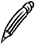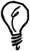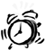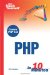# What Is an Array?

An array is a variable type that can store and index a set of values. An array is useful when the data you want to store has something in common or is logically grouped into a set.

#### Creating and Accessing Arrays

Suppose you wanted to store the average temperature for each month of the year. Using single-value variablesalso known as scalar variablesyou would need 12 different variables\$temp_jan, \$temp_feb, and so onto store the values. By using an array, you can use a single variable name to group the values together and let an index key indicate which month each value refers to.

The following PHP statement declares an array called \$temps and assigns it 12 values that represent the temperatures for January through December:

` \$temps = array(38, 40, 49, 60, 70, 79,                 84, 83, 76, 65, 54, 42); `

The array \$temps that is created contains 12 values that are indexed with numeric key values from 0 to 11. To reference an indexed value from an array, you suffix the variable name with the index key. To display March's temperature, for example, you would use the following:

` echo \$temps; `Index Numbers Because index values begin at zero by default, the value for Marchthe third monthis contained in the second element of the array.

The square brackets syntax can also be used to assign values to array elements. To set a new value for November, for instance, you could use the following:

` \$temps = 56; `Thearray Function The array function is a shortcut function that quickly builds an array from a supplied list of values, rather than adding each element in turn.

If you omit the index number when assigning an array element, the next highest index number will automatically be used. Starting with an empty array \$temps, the following code would begin to build the same array as before:

` \$temps[] = 38; \$temps[] = 40; \$temps[] = 49; ... `

In this example, the value 38 would be assigned to \$temps, 40 to \$temps, and so on. If you want to make sure that these assignments begin with \$temps, it's a good idea to initialize the array first to make sure there is no existing data in that array. You can initialize the \$temps array with the following command:

` \$temps = array(); `

#### Outputting the Contents of an Array

PHP includes a handy function, print_r, that can be used to recursively output all the values stored in an array. The following script defines the array of temperature values and then displays its contents onscreen:

` \$temps = array(38, 40, 49, 60, 70, 79,                 84, 83, 76, 65, 54, 42); print "<PRE>"; print_r(\$temps); print "</PRE>"; `

The <PRE> tags are needed around print_r because the output generated is text formatted with spaces and newlines. The output from this example is as follows:

` Array (      => 38      => 40      => 49      => 60      => 70      => 79      => 84      => 83      => 76      => 65      => 54      => 42 ) `print_r The print_r function can be very useful when you're developing scripts, although you will never use it as part of a live website. If you are ever unsure about what is going on in an array, using print_r can often shed light on the problem very quickly.

#### Looping Through an Array

You can easily replicate the way print_r loops through every element in an array by using a loop construct to perform another action for each value in the array.

By using a while loop, you can find all the index keys and their values from an arraysimilar to using the print_r functionas follows:

` while (list(\$key, \$value) = each(\$temps)) {   echo "Key \$key has value \$val <br>"; } `

For each element in the array, the index key value will be stored in \$key and the value in \$value.

PHP also provides another construct for traversing arrays in a loop, using a foreach construct. Whether you use a while or foreach loop is a matter of preference; you should use whichever you find easiest to read.

The foreach loop equivalent to the previous example is as follows:

` foreach(\$temps as \$key => \$value) {   ... } `Loops You may have realized that with the \$temps example, a for loop counting from 0 to 11 could also be used to find the value of every element in the array. However, although that technique would work in this situation, the keys in an array may not always be sequential and, as you will see in the next section, may not even be numeric.

#### Associative Arrays

The array examples so far in this chapter have used numeric keys. An associative array allows you to use textual keys so that the indexes can be more descriptive.

To assign a value to an array by using an associative key and to reference that value, you simply use a textual key name enclosed in quotes, as in the following examples:

` \$temps["jan"] = 38; echo \$temps["jan"]; `

To define the complete array of average monthly temperatures in this way, you can use the array function as before, but you indicate the key value as well as each element. You use the => symbol to show the relationship between a key and its value:

` \$temps = array("jan" => 38, "feb" => 40, "mar" => 49,               "apr" => 60, "may" => 70, "jun" => 79,               "jul" => 84, "aug" => 83, "sep" => 76,               "oct" => 65, "nov" => 54, "dec" => 42); `

The elements in an associative array are stored in the order in which they are defined (you will learn about sorting arrays later in this lesson), and traversing this array in a loop will find the elements in the order defined. You can call print_r on the array to verify this. The first few lines of output are as follows:

` Array (     [jan] => 38     [feb] => 40     [mar] => 49 ... `Sams Teach Yourself PHP in 10 Minutes
ISBN: 0672327627
EAN: 2147483647
Year: 2005
Pages: 151
Authors: Chris Newman

Similar book on Amazon

flylib.com © 2008-2017.
If you may any questions please contact us: flylib@qtcs.net